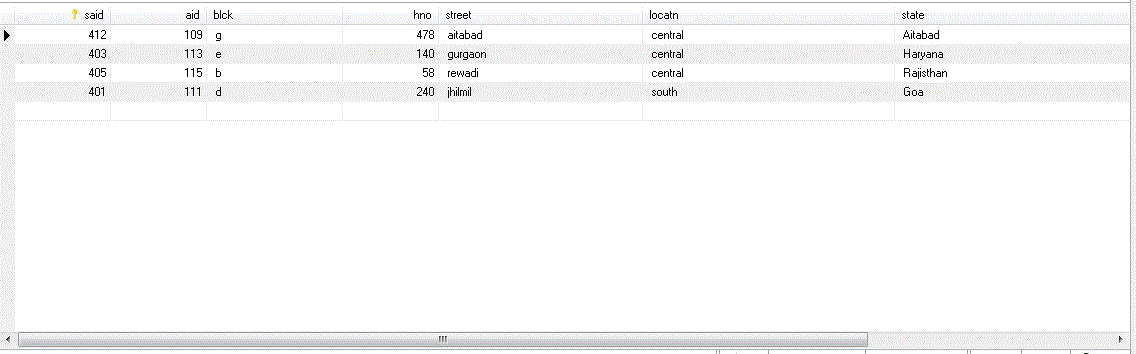# MySQL

Order By in MySQL
 Previous Home Next

In this clause we are sorting the table according to the specified order given. Default sorting order is ascending order in sorting of numeric values , where as in letters it follow alphabetical order.

### Syntax

```SELECT *
FROM tablename
ORDER BY value1, value2;
```

here some examples is given below

```SELECT *				//show all values
ORDER BY aid ;				//sorting numeric value default
```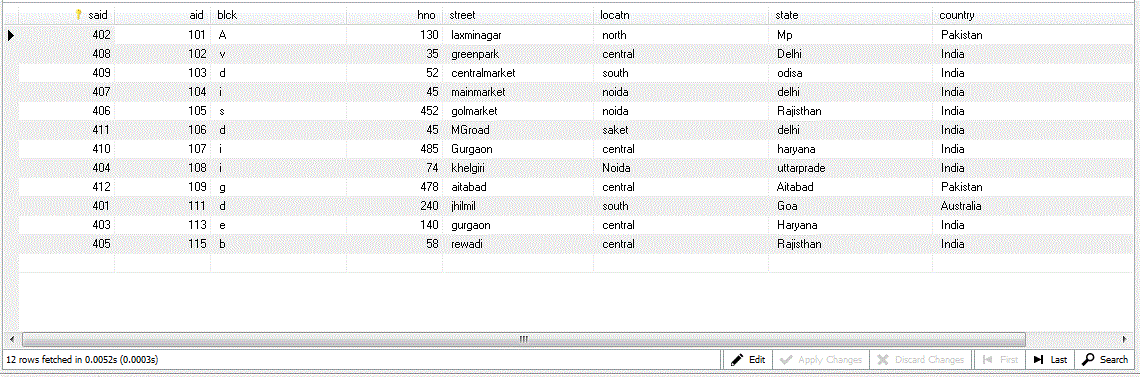### Example

```SELECT *				//show all values
ORDER BY aid desc ;			//sorting numeric value in descending order
```

Note Use DESC also used to reverse alphabetical to get the output in reverse order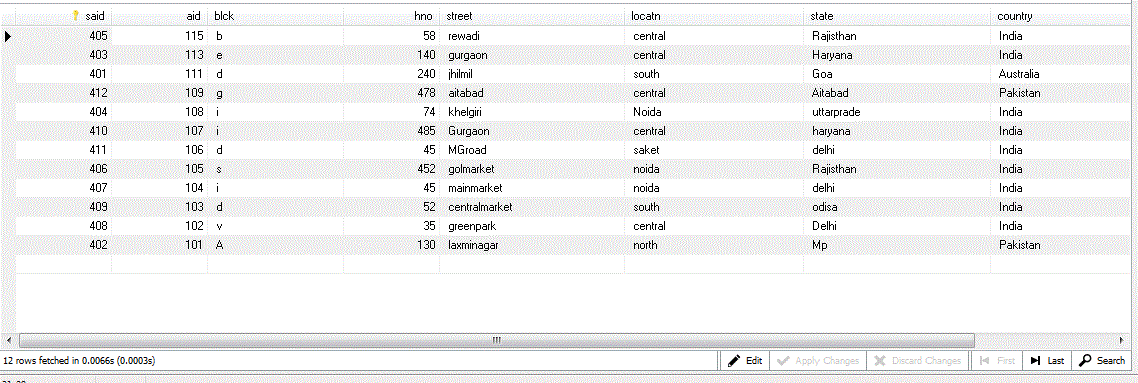### Example

```SELECT *					//show all values
ORDER BY locatn, state ;			//ordering order is given
```

Note Use locatn and state both to get the output in which order preferences is given to get the output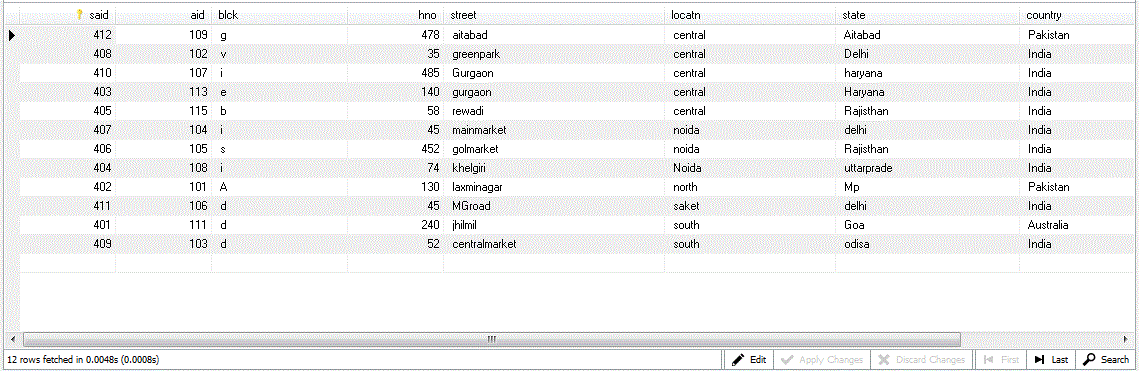### Example

```SELECT *					//show all values
WHERE aid > 108					//Condition can be use
ORDER BY aid;					//ordering order is given
```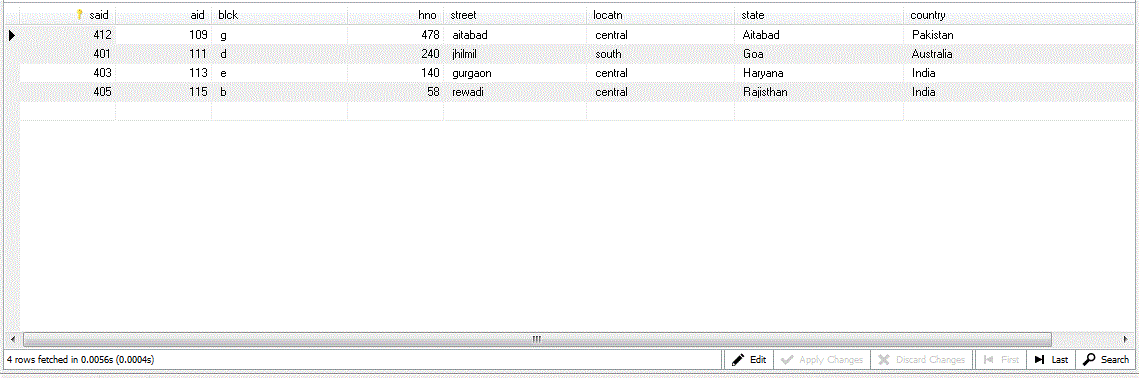### Example

```SELECT *					//show all values